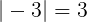# Java Math.abs() with Examples

Oct 12, 2019 · 3 mins readThe java.lang.Math class that comes bundled with Java contains various methods for performing basic numeric operations such as the elementary exponential, logarithm, square root, and trigonometric functions.

Math.abs(…) method is part of the Math class. It is used for finding the absolute value of a number. Absolute value is the magnitude of a real number without regard to its sign. If the argument is not negative, the argument is returned. If the argument is negative, the negation of the argument is returned.Math.abs(…) method is overloaded. Here are the method signatures:

``````public static int abs(int a)

public static long abs(long a)

public static float abs(float a)

public static double abs(double a)
``````

Note just like all other methods on the Math class, Math.abs(…) is a `static` method so you can call it directly on the Math class. The overloaded methods can take all primitive number types and the return type is the same as that of the argument.

``````int result = Math.abs(-3); // result = 3
``````

## Code Example

``````public class MathEx {
public static void main(String[] args) {
absolute();
}

private static void absolute() {
System.out.println(Math.abs(3)); // 3

System.out.println(Math.abs(-3)); // 3

System.out.println(Math.abs(1000L)); // 1000

System.out.println(Math.abs(2.0f)); // 2.0

System.out.println(Math.abs(-2.0f)); // 2.0

System.out.println(Math.abs(-0)); // 0

System.out.println(Math.abs(Double.NEGATIVE_INFINITY)); // + Infinity
}
}
``````

Output

``````3
3
1000
2.0
2.0
0
Infinity
``````

There you have it. Hope you found this tutorial useful!

### Special cases

For floating-point numbers i.e. `float` or `double`:

• If the argument is positive zero or negative zero, the result is positive zero.
• If the argument is infinite, the result is positive infinity.
• If the argument is NaN, the result is NaN.

If you need more detailed information, please see Javadocs. It’s worth noting that the `Math` class in Java contains several other useful methods for arithmetic, logarithms and trignometric operations.

``````<iframe width="560" height="315" src="https://www.youtube.com/embed/JzMdepMLW44" frameborder="0" allow="accelerometer; autoplay; encrypted-media; gyroscope; picture-in-picture" allowfullscreen></iframe>